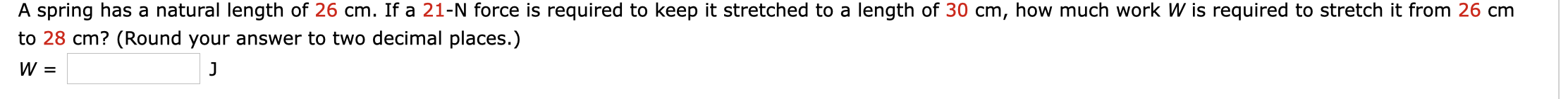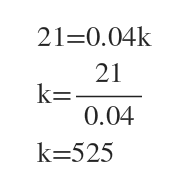# A spring has a natural length of 26 cm. If a 21-N force is required to keep it stretched to a length of 30 cm, how much work W is required to stretch it from 26 cmto 28 cm? (Round your answer to two decimal places.)

Question
23 viewshelp_outlineImage TranscriptioncloseA spring has a natural length of 26 cm. If a 21-N force is required to keep it stretched to a length of 30 cm, how much work W is required to stretch it from 26 cm to 28 cm? (Round your answer to two decimal places.) fullscreen
check_circle

star
star
star
star
star
1 Rating
Step 1

We know that F(x)=kx , where k=constant.

From 26 cm to 30 cm , distance is (30-26)=4 cm = 0.04 m

For x= 0.04m , force needed = 21 N

Using this we find k.So, F(x)= 525x

...

### Want to see the full answer?

See Solution

#### Want to see this answer and more?

Solutions are written by subject experts who are available 24/7. Questions are typically answered within 1 hour.*

See Solution
*Response times may vary by subject and question.
Tagged in